Newton’s laws of motionPage 5

WATCH ALL SLIDES

A. less than 50 N.

50 N.

more than 50 N.

None of the above.

Newton’s Second Law of Motion

Slide 35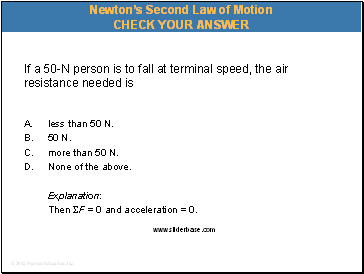If a 50-N person is to fall at terminal speed, the air resistance needed is

A. less than 50 N.

50 N.

more than 50 N.

None of the above.

Explanation:

Then F = 0 and acceleration = 0.

Newton’s Second Law of Motion

Slide 36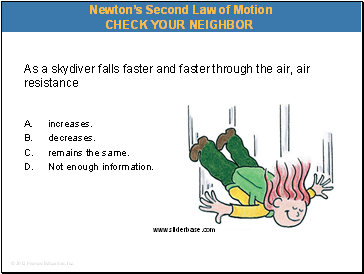As a skydiver falls faster and faster through the air, air resistance

A. increases.

decreases.

remains the same.

Not enough information.

Newton’s Second Law of Motion

Slide 37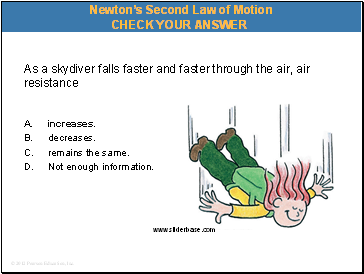As a skydiver falls faster and faster through the air, air resistance

A. increases.

decreases.

remains the same.

Not enough information.

Newton’s Second Law of Motion

Slide 38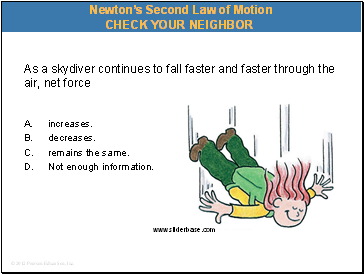As a skydiver continues to fall faster and faster through the air, net force

A. increases.

decreases.

remains the same.

Not enough information.

Newton’s Second Law of Motion

Slide 39As a skydiver continues to fall faster and faster through the air, net force

A. increases.

decreases.

remains the same.

Not enough information.

Newton’s Second Law of Motion

Slide 40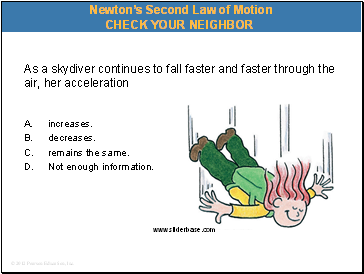As a skydiver continues to fall faster and faster through the air, her acceleration

A. increases.

decreases.

remains the same.

Not enough information.

Newton’s Second Law of Motion

Slide 41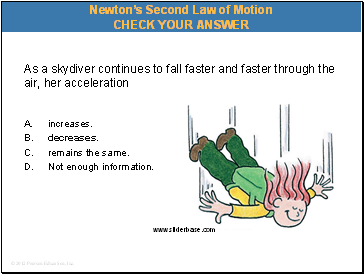As a skydiver continues to fall faster and faster through the air, her acceleration

A. increases.

decreases.

remains the same.

Not enough information.

Newton’s Second Law of Motion

Slide 42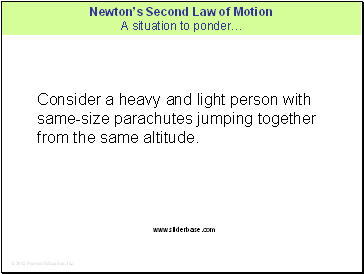Newton’s Second Law of Motion A situation to ponder…

Consider a heavy and light person with same-size parachutes jumping together from the same altitude.

Slide 43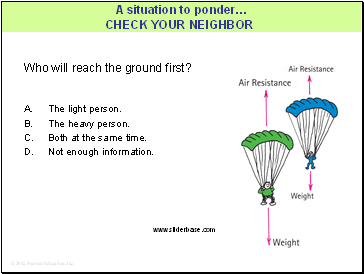Who will reach the ground first?

A. The light person.

The heavy person.

Both at the same time.

Not enough information.

A situation to ponder…